# Liquidity Ratio

Liquidity means the firms ability to meet its current liabilities. In other words, the ability of a business to pay its short-term debts is frequently referred to as liquidity position of the business. Short term creditors of the firm are generally interested to know about the liquidity position of the firm. The liquidity ratio is further categorised into two parts: (i) Current Ratio & (ii) Liquid Ratio

a. Current Ratio : It shows the relationship between current assets and current liabilities. It is also known as working capital ratio. The ideal current ratio is 2:1.  The term current assets means the assets which are easily convertible into cash or cash equivalents within 12 months & the term current liabilities means the liabilities which are payable within a period of 12 months.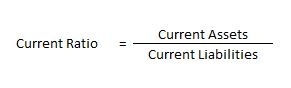b. Liquid Ratio : It shows the relationship between liquid assets and current liabilities. It is also known as acid test ratio or quick ratio. The ideal liquid ratio is 1:1.  The term liquid assets means the assets which are easily convertible into cash or cash equivalents very shortly. All current assets except inventories and prepaid expenses are included in liquid assets.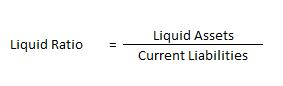### How to compute current ratio – Liquidity Ratio – Question 1 : –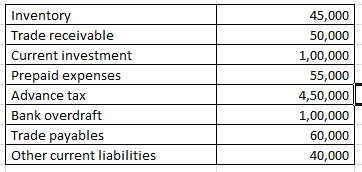On the basis of the following information calculate the current ratio:

#### Explanation : –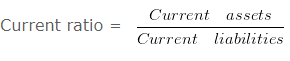Current ratio = 700000/200000

Current ratio = 3.5 :1
Working note 1 : Current assets = Inventory + Trade receivable + Current investment + Prepaid expenses + Advance tax
Current assets = 45000 + 50000 + 100000 + 55000 + 450000
Current assets = 700000
Working note 2 : Current liabilities = Bank overdraft + Trade payables + Other current liabilities
Current liabilities = 100000 + 60000 + 40000
200000

### How to compute liquidity ratio – Question 2

On the basis of the following information calculate the liquidity ratio: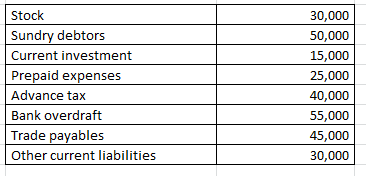#### Explanation : –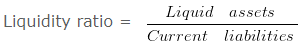Liquidity ratio = 65000/130000

Liquidity ratio = 0.5 :1
Working note 1 : Liquid assets = Current assets (-) ( Stock + Prepaid expenses + Advance tax )
Liquid assets = 160000 (-) 30000 + 25000 + 40000
Liquid assets = 65000
Working note 2 : Current assets = Stock + Sundry debtors + Current investment + Prepaid expenses + Advance tax
Current assets = 30000 + 50000 + 15000 + 25000 + 40000
Current assets = 160000
Working note 3 : Current liabilities = Bank overdraft + Trade payables + Other current liabilities
Current liabilities = 55000 + 45000 + 30000
130000

### How to compute quick ratio – Liquidity Ratio – Question 3

On the basis of the following information calculate the Quick ratio:

#### Explanation : –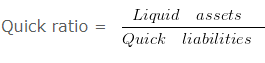Quick ratio = 21000/12000

Quick ratio = 1.75 :1
Working note 1 : Liquid assets = Current assets (-) ( Inventory + Prepaid expenses + Advance tax )
Liquid assets = 65000 (-) 20000 + 18000 + 6000
Liquid assets = 21000
Working note 2 : Quick liabilities = Current liabilities (-) Bank overdraft
Quick liabilities 32000 (-) 20000
Quick liabilities 12000
Working note 3 : Current assets = Inventory + Trade receivable + Current investment + Prepaid expenses + Advance tax
Current assets = 20000 + 15000 + 6000 + 18000 + 6000
Current assets = 65000
Working note 4 : Current liabilities = Bank overdraft + Trade payables + Other current liabilities
Current liabilities = 20000 + 5000 + 7000
32000

### When current liabilities are given – Question 4

From the following information compute the current ratio.

#### Explanation : –Current Ratio = 1300000/400000

Current Ratio = 3.25 :1
Workings:
Working Capital = Current assets (-) current liabilities
900000 = Current assets (-) 400000
Current assets = 1300000
Workings:
current liabilities = Trade Payables (+) Other Current Liabilities
current liabilities = 225000 (+) 175000
current liabilities = 400000

### Determination of working capital – Liquidity Ratio – Question 5

From the following given information:
A firm had current assets of RS. 140000
it then paid a current liability of RS. 20000
After this payment the current ratio was 3 : 2
Determine the current liabilities and working capital after the payment.

#### Explanation : –

Working capital = Current assets (-) Current liabilities
= 120000 (-) 80000
= 40000
Workings:
Current Assets = 140000
Current Assets After the payment of RS. 20000 would be 140000 (-) 20000 = 120000
As current ratio is 3 : 2 and current assets are RS. 1200003/2 = 120000/Current liabilities

Current liabilities = 240000/3

Current liabilities = 80000

### Determination of working capital when current ratio given – Question 6

From the following information:
A firm had current assets of RS. 225000
it then paid a current liability of RS. 45000
After this payment the current ratio was 9 : 7
Determine the current liabilities and working capital Before the payment.

#### Explanation : –

(i) Working capital = Current assets (-) Current liabilities
= 180000 (-) 140000
= 40000
Workings:
Current Assets = 225000
Current Assets After the payment of RS. 45000 would be 225000 (-) 45000 = 180000
As current ratio is 9 : 7 and current assets are RS. 1800009/7 = 180000/Current liabilities

Current liabilities = 1260000/9

Current liabilities = 140000
Before the payment of liabilities of RS. 45000 total amount of
Current liabilities = 45000 + 140000
Current liabilities = 185000
Working capital = 40000

### Liquid ratio on the basis of working capital – Liquidity Ratio – Question 7

Current Assets = Rs. 80000 . Inventory = Rs. 15000 . Prepaid Expenses = Rs. 50000 . Working Capital = Rs. 50000 Calculate Liquid Ratio.

#### Explanation : –

Liquid Assets = Current Assets (-) Inventory (-) Prepaid Expenses
Liquid Assets = 80000 (-) 15000 (-) 50000
Liquid Assets = 15000
Current Liabilities = 30000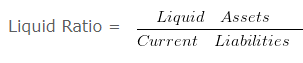Liquid Ratio = 15000/30000

Liquid Ratio = 0.5
Working Note 1 :
Current Liabilities = Current Assets (-) Working Capital
= 80000 (-) 50000
= 30000

### Calculation of Liquid Ratio – Question 8

Current Assets of ABC Ltd. are Rs. 72000 and the current ratio is 1.2 . Value of inventories is Rs. 27000 . Calculate liquid ratio.

#### Explanation : –OR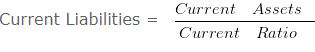Current Liabilities = 72000/1.2

Current Liabilities = 60000Quick Ratio = 45000/60000

Quick Ratio = 0.75
Working Notes:
Liquid Assets = Current Assets (-) Inventory
Liquid Assets = 72000 (-) 27000
Liquid Assets = 45000

### Current Ratio on the basis of Quick Ratio – Liquidity Ratio – Question 9

Value of Inventory of HRD & Co. is Rs. 240000 . Liquid Assets are Rs. 120000
Quick Ratio is 0.5 . Calculate the current ratio .

#### Explanation : –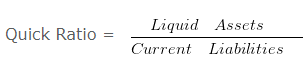OR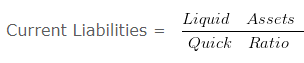Current Liabilities = 120000/0.5

Current Liabilities = 240000Current Ratio = 360000/240000

Current Ratio = 1.5 :1
Working Notes:
Current Assets = Liquid Assets + Inventory
= 120000 + 240000
= 360000